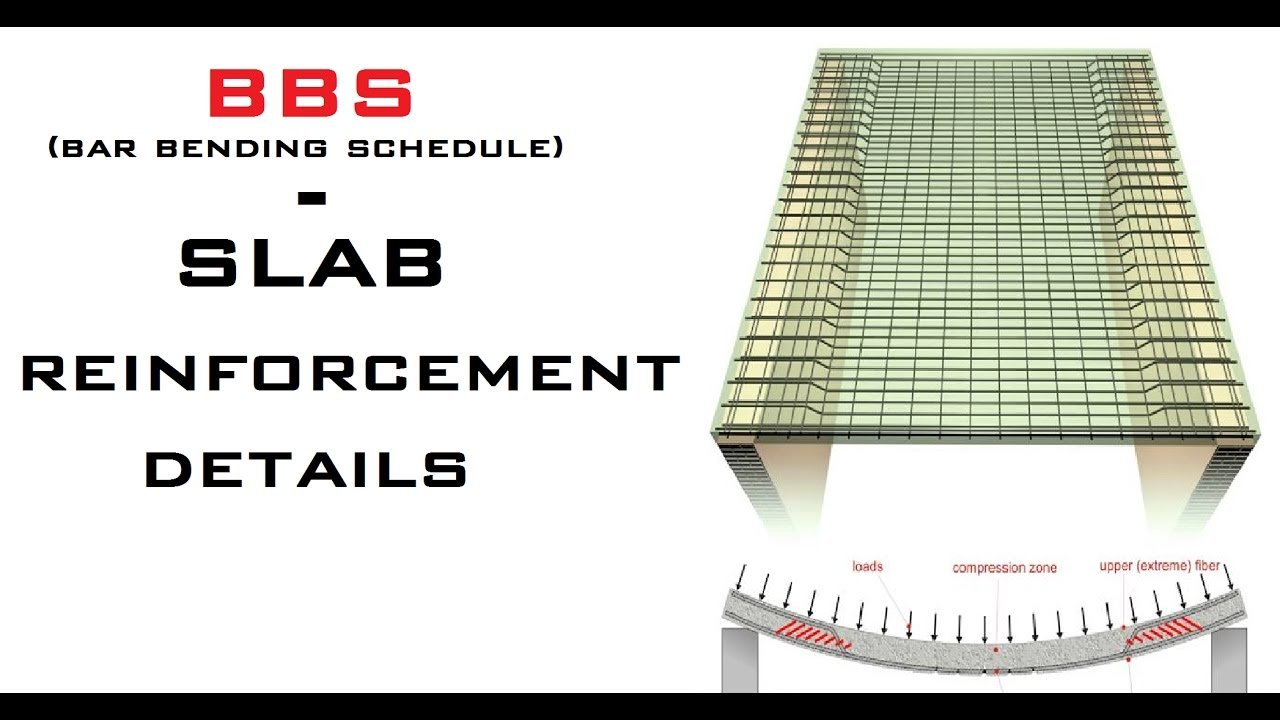Breaking News
Home / Civil Engineering / Bar Bending Schedule / How to Calculate the Unit Weight of Steel Bars | Bar Bending Schedule

# How to Calculate the Unit Weight of Steel Bars | Bar Bending Schedule

Cutting Length of Spiral Bar or Helix Bar | Bar Bending Schedule, commonly referred to as “BBS” is a comprehensive list that describes the location, mark, type, size, length and number, and bending details of each bar or fabric in a Reinforcement Drawing of a Structure.

This process of listing the location, type and size, number of, and all other details is called “Scheduling”. In the context of Reinforcement bars, it is called the bar schedule. In short, Bar Bending Schedule is a way of organizing rebars for each structural unit, giving detailed reinforcement requirements.

# How to Calculate the Unit Weight of Steel BarsAfter estimating it is very important to know the unit weight of steel bars because, we estimate as 100 meter 20mm ø bar or 100 feet 16mm ø bar, etc (ø is the symbol of diameter).

But steel bar suppliers will not understand this notation, they measure the steel bars in weight. So we have to order them in kg or quintal or ton. In this article, we will discuss how to calculate unit weight of steel bars of different diameters.

The formula is W = D²L/162

Where

W = Weight of steel bars.

D = Diameter of steel bars in mm.

L = Length of bars in meter.

Example 1: Calculate the weight of 60 meters long 12 mm ø bar.

Here, D = 12 mm.

L= 60 m.

We know that, W = D²L/162

W = 12² x 60/162 = 53 kg

Weight of 60 m 12mm ø bar is 53 kg.

Let’s look for another example.

### Column Reinforcement Detail | Lateral Reinforcement for Columns | Steel Reinforcement

Example 2: Calculate the weight of 100 m 16 mm ø bar.

Here, D = 16 mm.

L = 100 m.

W = 16² x 100/162 = 158 kg.

If we put 1-meter length for each diameter of the steel bar in the formula then we will get the unit weight.

• 10mm ø bar = 10² x 1/162 = 0.617 kg/m
• 12mm ø bar = 12² x 1/162 = 0.888 kg/m
• 16mm ø bar = 16² x 1/162 = 1.580 kg/m
• 20mm ø bar = 20² x 1/162 = 2.469 kg/m

If we multiply the length of bars with this unit weight we will get the total weight of steel bars.

For example, the total weight of 1000 meter long 20mm ø steel bar is,

1000 x 2.469 = 2469 kg.

Using the same method we can calculate the unit weight of different steel bars.

Here I have calculated in meter but we can also calculate in foot. To calculate in foot we have to use the following formula:

W= D²L/533

Where D = Diameter of bars in mm.

L = Length of bars in foot.

### THANKS.